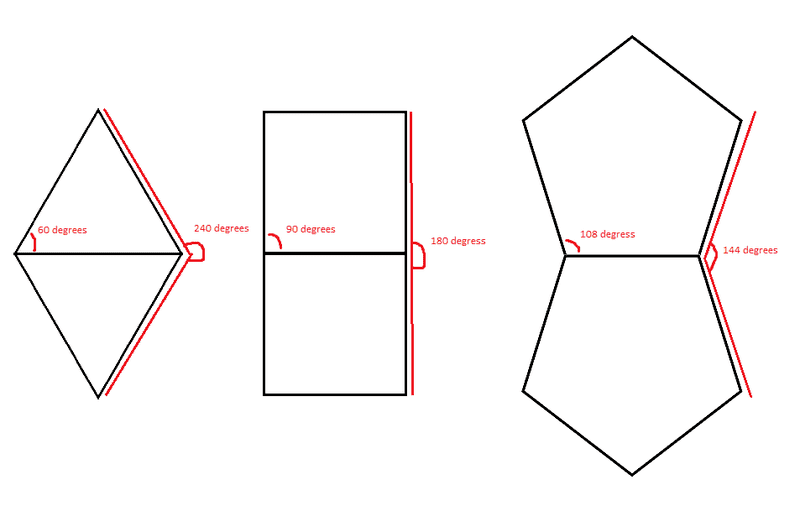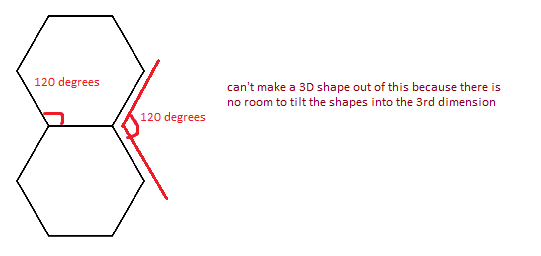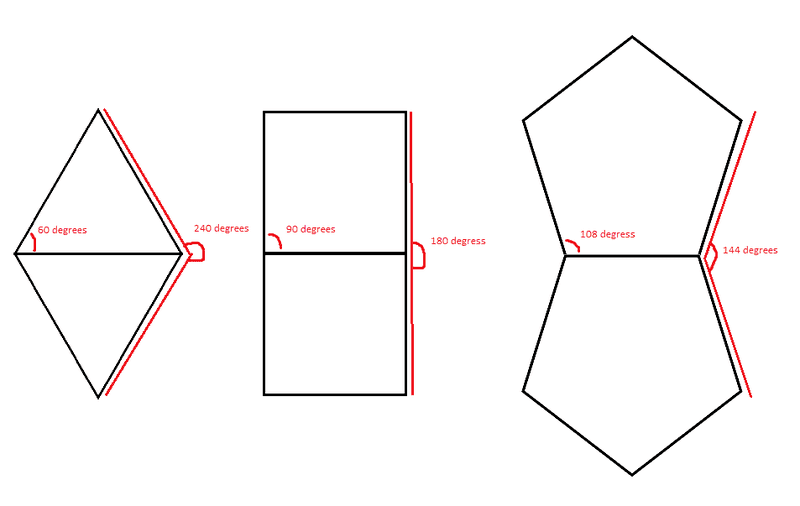# What's it called when a 3D shape can be made of 2D surfaces of all the same size

What's it called when a 3D shape can be made of 2D surfaces of all the same shape and dimensions?

To make a cube, I can use 6 4-sided-squares (of course they're 4 sided)
To make a pyramid (3 sided), I can use 4 3-sided-triangles

I can do this with pentagon as well (i don't know what the shape is called)

Eventually I can't do this as the sides of the shape increase because the remaining angle.

... after some thinking I realized I can only do this with 3 equilateral shapes.
3 sided
4 sided
and 5 sidedOnce I get to the hexagon ... wellNow here's an even more interesting question: Are there any others shapes convex or concave equilateral or not that can be used to create a 3D shape? (where the base shapes remains the same)
If so how can one determine if a shape is capable of doing this?

turbo
Gold Member
Regular polyhedron

Thanks!

Doesn't look like there are too many of those shapes.

Do you know about the second questions?
Now here's an even more interesting question: Are there any others shapes convex or concave equilateral or not that can be used to create a 3D shape? (where the base shapes remains the same)
If so how can one determine if a shape is capable of doing this?

What's it called when a 3D shape can be made of 2D surfaces of all the same shape and dimensions?

To make a cube, I can use 6 4-sided-squares (of course they're 4 sided)
To make a pyramid (3 sided), I can use 4 3-sided-triangles

I can do this with pentagon as well (i don't know what the shape is called)

Eventually I can't do this as the sides of the shape increase because the remaining angle.

... after some thinking I realized I can only do this with 3 equilateral shapes.
3 sided
4 sided
and 5 sidedOnce I get to the hexagon ... wellNow here's an even more interesting question: Are there any others shapes convex or concave equilateral or not that can be used to create a 3D shape? (where the base shapes remains the same)
If so how can one determine if a shape is capable of doing this?

You can use the Euler Formula to prove, if I remember correctly, there are only five which are all regular polygons. 4,6,8,12,20